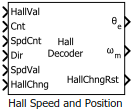# Hall Speed and Position

Compute speed and estimate position of rotor by using Hall sensors

• Library:
• Motor Control Blockset / Sensor Decoders

•## Description

The Hall Speed and Position block computes the mechanical speed of the rotor by tracking changes in the Hall state. The block also estimates the electric position of the rotor by using the direction, Hall state, and external counter value inputs.

The block executes periodically after a fixed time interval that the controller algorithm defines.

## Ports

### Input

expand all

The Hall state at current time. For example, these are the possible valid Hall states (where the MSB represents the output of the first Hall sensor connected):

• 5 - (101)

• 4 - (100)

• 6 - (110)

• 2 - (010)

• 3 - (011)

• 1 - (001)

Data Types: `single` | `double` | `fixed point`

The external counter value that the block uses to determine the time elapsed between the Hall state change and block execution.

Data Types: `single` | `double` | `fixed point`

This value indicates the clock cycles (time) elapsed between two consecutive changes in the Hall state.

Data Types: `single` | `double` | `fixed point`

The direction of the rotor spin (either +1 or –1 indicating positive or negative direction of rotation, respectively) during the current Hall state.

Data Types: `single` | `double` | `fixed point`

The port value indicates Hall state validity. The value zero indicates that the current or previous Hall state is invalid and that the block cannot calculate speed and position.

The value one indicates that both the current and previous Hall states are valid and that the block can calculate speed and position.

Data Types: `single` | `double` | `fixed point`

The port value indicates Hall state change and block execution status. The value one indicates that the Hall state changed, but that the block execution is pending. The value zero indicates that the block has completed executing the last Hall state change.

Data Types: `single` | `double` | `fixed point`

### Output

expand all

The estimated electrical position of the rotor based on the Expected hall sequence in positive direction parameter and the `Direction`, `HallVal`, and `CounterValue` inputs.

#### Dependencies

To enable this port, set Block output to either `Position` or ```Speed and position```.

Data Types: `single` | `double` | `fixed point`

The mechanical speed of the rotor in revolutions per minute. The block calculates this value by tracking the Hall state changes.

The port returns zero if the `SpdVal` input is zero.

#### Dependencies

To enable this port, set Block output to either `Speed` or ```Speed and position```.

Data Types: `single` | `double` | `fixed point`

The port outputs a value of zero (sets the Hall state change flag to zero) indicating that the block has successfully executed speed and position computations for the last Hall state change.

Data Types: `single` | `double` | `fixed point`

## Parameters

expand all

### General

Select the available block output ports as one of the following values:

• Speed and position

• Speed

• Position

The register size of the external counter. The maximum counter value is ${\text{2}}^{\text{n}}\text{-1}$, where $\text{n}$ = counter size.

The clock frequency of the external counter.

The time between two consecutive instances of block execution.

Number of pole pairs available in the motor.

The block does not calculate position for speed below this value.

Rotor angular displacement that represents the interval at which the `SpdCnt` port value was calculated.

Unit of the angular velocity or mechanical speed (`⍵m`) output.

#### Dependencies

To enable this parameter, set Block output to either `Speed` or ```Speed and position```.

Data type of the rotor angular speed output.

#### Dependencies

To enable this parameter, set Block output to either `Speed` or ```Speed and position```.

### Position

The Hall sensor sequence that represents the positive direction of rotor spin.

#### Dependencies

To enable this parameter, set Block output to either `Position` or ```Speed and position```.

The custom sequence that you can enter to represent rotor spin in the positive direction.

#### Dependencies

To enable this parameter:

• Set Block output to either `Position` or ```Speed and position```.

• Set Expected hall sequence in positive direction to `Custom sequence`.

The `1st Order` option is less accurate in computing position, but quick. The `2nd Order` option is more accurate, but needs more computation time. These equations describe the options:

where:

= Position computed by using 1st order extrapolation.

= Position computed by using 2nd order extrapolation.

${\theta }_{sector}$ = Sector angle defined by the Hall sensor output.

$\omega$ = Angular velocity of the rotor.

$\alpha$ = Angular acceleration of the rotor.

$t$ = Time spent in a sector.

#### Dependencies

To enable this parameter, set Block output to either `Position` or ```Speed and position```.

Unit of angular speed output.

#### Dependencies

To enable this parameter, set Block output to either `Position` or ```Speed and position```.

Data type of angular speed output.

#### Dependencies

To enable this parameter, set Block output to either `Position` or ```Speed and position```.

## Version History

Introduced in R2020a